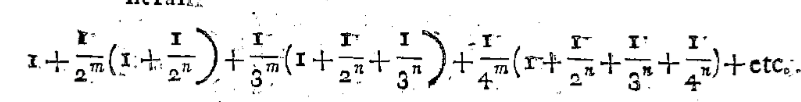# What did Euler do with multiple zeta values?

When reading about multiple zeta values, I often find the claim that the case of length two $$\zeta(s_1, s_2)=\sum_{n>m \geq 1} \frac{1}{n^{s_1}m^{s_2}}, \qquad s_1 \geq 2, \quad s_2 \geq 1$$ was first considered by Euler. Does anybody know what precisely he proved about these numbers?

• This may fit better at hsm.stackexchange.com Feb 2, 2016 at 16:02
• He was trying to understand $\zeta(3)$ after he discovered the rationality of $\zeta(2k)/\pi^{2k}$. He was unsuccessful with $\zeta(3)$ but was led to many identities among MZV (which arise naturally), the simplest being $\zeta(3) = \zeta(2,1)$. See the introduction (pages 11-13) of Bombieri's article The classical theory of zeta and $L$-functions in the Milan Journal of Mathematics. Feb 2, 2016 at 16:20

Euler proved in 1775 in Meditationes circa singulare serierum genus ("Meditations about a singular type of series") that $$\sum_{i+j=n,\,i\geq 2,\;j\geq 1}\zeta(i,j)=\zeta(n),$$ as a special case of a more general sum rule.
Notice that Euler's definition of the multiple-zeta-value is slightly different than in the OP ($$n\geq m$$ instead of $$n>m$$)